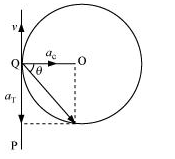## Pages

### Motion in a plane NCERT Solutions Class 11 Physics - Solved Exercise Question 4.31

Question 4.31:

A cyclist is riding with a speed of 27 km/h. As he approaches a circular turn on the road of radius 80 m, he applies brakes and reduces his speed at the constant rate of 0.50 m/s every second. What is the magnitude and direction of the net acceleration of the cyclist on the circular turn ?

Solution:

0.86 m/s2; 54.46° with the direction of velocity
Speed of the cyclist, v = 27 km/h = 7.5 m/s
Radius of the circular turn, r = 80 m
Centripetal acceleration is given as:
ac = v2 / r
= (7.5)2 / 80 = 0.7 ms-2

The situation is shown in the given figure:Suppose the cyclist begins cycling from point P and moves toward point Q. At point Q, he applies the breaks and decelerates the speed of the bicycle by 0.5 m/s2.
This acceleration is along the tangent at Q and opposite to the direction of motion of the cyclist.
Since the angle between ac and aT is 900, the resultant acceleration a is given by:
a = (ac2 + aT2)1/2
= ( (0.7)2 + (0.5)2 )1/2
= (0.74)1/2 = 0.86 ms-2
tan θ = ac / aT
where θ is the angle of the resultant with the direction of velocity.
tan θ = 0.7 / 0.5 = 1.4
θ = tan-1 (1.4) = 54.560